## Tuesday, November 19, 2013

### Understanding inferential statistics using correlation example

Understanding inferential statistics using correlation example

# Understanding inferential statistics using correlation example

## Introduction

In the following R and knitr experiment/blog post I will be documenting my play with correlation and inferences. I am just reading Discovering Statistics Using R by Andy Field and I am trying to code some staff from the book, plus experiment and see how inferential statistis work.

Simulations are great way to learn statistics in my opinion and in opinion of Will Hopkins. I hope that someone might find this blog post intresting and learn a thing or two.

As I have pointed out in previous blog posts, sport coaches are not interested in inferential statistics, but rather individual reaction/effects, yet most if not all research utilize inferential statistics. Why is that? Because in research we are interested in effects overall (or on average) on a given population, and not on a single individual or sample. In research, subjects are just vehicles, a way to get numbers/estimates or obeservations, while in sport they are what matters the most.

Since it very hard to measure the whole population, we need to make inferences from smaller sample to the bigger population. To do this we use Central Limit Theorem and estimated standard error (it is beyond me why standard error is not called sampling error, because it conveys much more meaning).

Understanding this of crucial importance to understand statistics and I have struggled with this mainly because most books don't put much pages/emphasis on getting it and jump to ANOVAs and all thet fancy stuff too soon.

Enough of my rant - I hope that this blog post might yield some light on population/sample inferences for the students. I will use correlation as an estimate we are interested into (it could be mean, SD, Cohen's effect size, whatever - the idea is the same).

## Population correlation

Creating population with two estimates that correlate - in this case squat and vertical jump in athletes (NOTE: All data are imaginary for the sake of an example)

``````populationSize <- 10000

# Simulate vertical jump and squat estiamtes in population
randomError = 8
populationSquatKG <- rnorm(populationSize, mean = 150, sd = 10)
populationVerticalJumpCM <- populationSquatKG * 0.45 - 20 + rnorm(populationSize,
mean = 0, sd = randomError)

# Graph the populations and scatter
par(mfrow = c(1, 3))

hist(populationSquatKG, 30, col = "blue", xlab = "kg", main = "Squat 1RM in kg")

hist(populationVerticalJumpCM, 30, col = "yellow", xlab = "cm", main = "Vertical Jump Height in cm")

plot(populationSquatKG, populationVerticalJumpCM, col = "grey", main = "Scatterplot between Squat \nand Vertical Jump",
xlab = "Squat 1RM in kg", ylab = "Vertical Jump Height in cm")

# Add Text (r=) on the graph
text(min(populationSquatKG) * 1.1, max(populationVerticalJumpCM) * 0.9, paste("r=",
as.character(round(cor(populationSquatKG, populationVerticalJumpCM), 2)),
sep = ""), cex = 1.5)
``````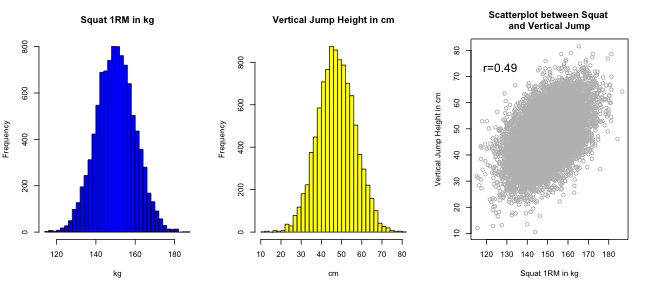In the population above r=0.49 between vertical jump and squat. Let's see what happens with correlation when we modify the random error parameter.

``````par(mfrow = c(3, 4))

for (randomError in seq(from = 0, to = 30, length.out = 12)) {

popVerticalJumpCM <- populationSquatKG * 0.45 - 20 + rnorm(populationSize,
mean = 0, sd = randomError)

# Graph the scatter
plot(populationSquatKG, popVerticalJumpCM, col = "grey", xlab = "Squat 1RM in kg",
ylab = "Vertical Jump Height in cm", main = paste("r= ", as.character(round(cor(populationSquatKG,
popVerticalJumpCM), 2)), "\nRandom Error= ", as.character(round(randomError,
2)), sep = ""))

}
``````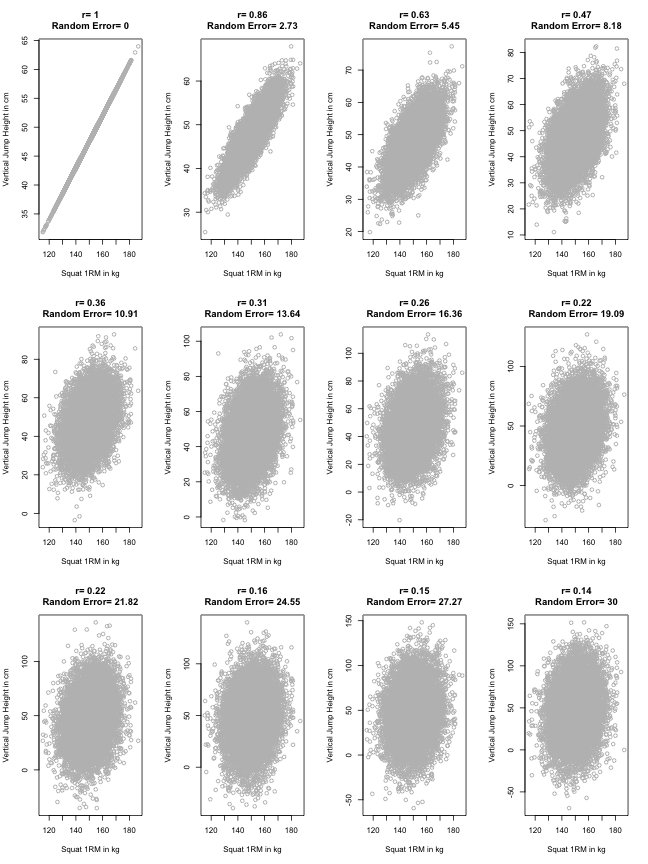As can be seen from the graphs above the higher the random error the lower the correlation.

## Sample correlation

Let's get back to our original population where r=0.49 between vertical jump and squat. Because we can't measure the whole population (practically), we need to take a small random sample from the population (N=50).

``````# Put our population squat and vertical jump into data frame for easier
# sampling
population <- data.frame(squat = populationSquatKG, verticalJump = populationVerticalJumpCM)

# Take a sample from the population
sampleSize <- 50

sample <- population[sample(nrow(population), sampleSize), ]

# Graph the sample histogram and scatter
par(mfrow = c(1, 3))

hist(sample\$squat, 10, col = "blue", xlab = "kg", main = "Sample Squat 1RM in kg")

hist(sample\$verticalJump, 10, col = "yellow", xlab = "cm", main = "Sample Vertical Jump Height in cm")

plot(sample\$squat, sample\$verticalJump, col = "grey", main = "Scatterplot between sample Squat \nand Vertical Jump",
xlab = "Squat 1RM in kg", ylab = "Vertical Jump Height in cm")

# Add Text (r=) on the graph
text(min(sample\$squat) * 1.1, max(sample\$verticalJump) * 0.9, paste("r=", as.character(round(cor(sample\$squat,
sample\$verticalJump), 2)), sep = ""), cex = 1.5)
``````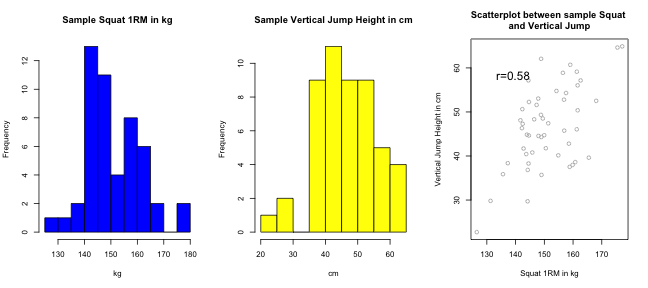What you will notice is that correlation in the whole population (N=104) r=0.49 differs from the correlation in the sample (N=50) r=0.58. What we are interested in are making inferences to a whole population from a small sample. As you can see they are not the same, so how can we be certain what is the correlation in the population from drawing a small sample?

## Sampling distribution

What would happend if we repeat the sampling a lot of times? Let's try out and let's plot the sampling distribution of the sample correlation (N=50).

``````samplingNumber <- 5000

sampleCorrelation <- rep(0, samplingNumber)

for (i in seq(from = 1, to = samplingNumber)) {
# take sample from population
sample <- population[sample(nrow(population), sampleSize), ]

# remember the correlation
sampleCorrelation[i] = cor(sample\$squat, sample\$verticalJump)
}

# Plot the histogram of sampling correlations
hist(sampleCorrelation, 50, col = "blue", main = "Sampling distribution of the sample correlation",
xlab = "Correlation", xlim = c(0, 1.2))

# Plot the line representing correlation in the population
abline(v = cor(populationSquatKG, populationVerticalJumpCM), col = "red", lwd = 3)
``````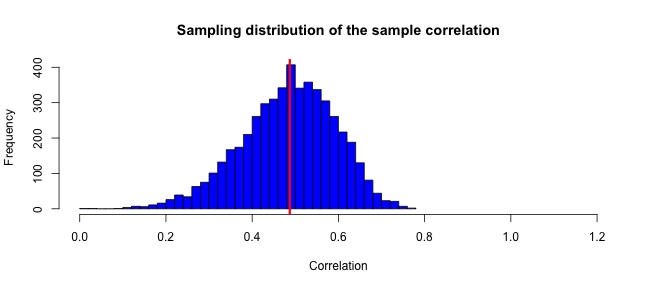What is noticable is that by repeating sampling most of our sample correlations are distributed around population correlation (red line). The “spread” around mean (correlation in population) is expressed with standard deviation and it is called standard error (SE).

Interestingly enough, standard error (or standard deviation of sampling distribution of the sample correlation; yeah a mouthful) depends on the sample size. The larger the sample size (N) the lower the standard error (SE) and vice versa. Let's simulate it and plot it.

``````
samplingNumber <- 5000

sampleCorrelation <- rep(0, samplingNumber)

par(mfrow = c(4, 3))
for (sampleSize in seq(from = 10, to = 120, length.out = 12)) {

for (i in seq(from = 1, to = samplingNumber)) {
# take sample from population
sample <- population[sample(nrow(population), sampleSize), ]

# remember the correlation
sampleCorrelation[i] = cor(sample\$squat, sample\$verticalJump)
}
# Plot the histogram of sampling correlations
hist(sampleCorrelation, 50, col = "blue", main = paste("N=", sampleSize,
"\nSE=", round(sd(sampleCorrelation), 2)), xlab = "Correlation", xlim = c(0,
1.2))

# Plot the line representing correlation in the population
abline(v = cor(populationSquatKG, populationVerticalJumpCM), col = "red",
lwd = 3)
}
``````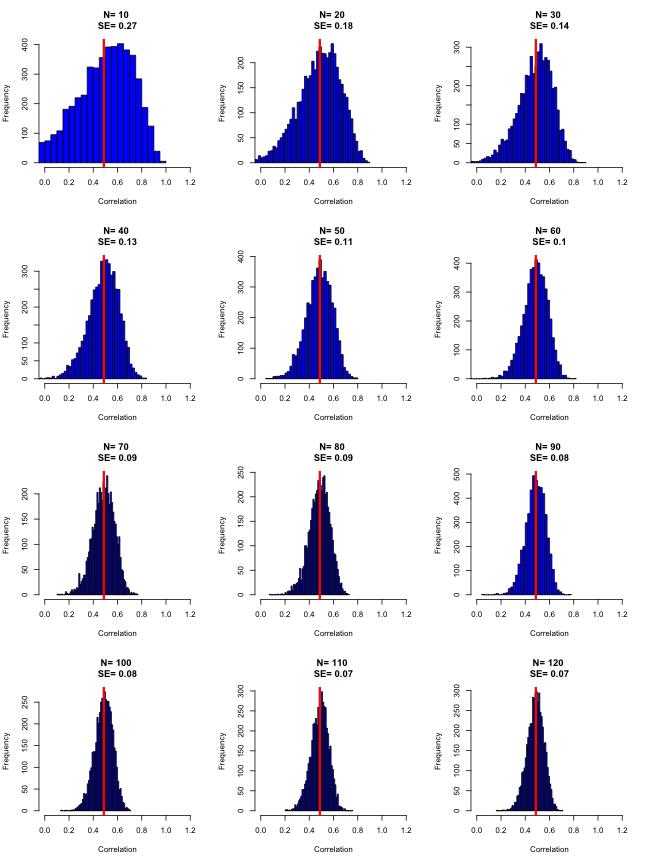As can be seen from the graph above the larger the sample size the smaller the standard error (SE).

Before we can proceed with how to use SE to make inferences, we must deal with one assumption to make this work. For all inferential tests in statistics there must be couple of assumptions met - one of the most important one is the assumption of normality. One problem with sampling distribution of the sample correlation is that it is not normally distributed. Hence, correlation coefficient have to be adjusted. I am not going into that formula here, but I will compare sampling distribution of unadjusted and adjuster correlation using sample size N=50

``````samplingNumber <- 5000
sampleSize <- 50

sampleCorrelation <- rep(0, samplingNumber)

for (i in seq(from = 1, to = samplingNumber)) {
# take sample from population
sample <- population[sample(nrow(population), sampleSize), ]

# remember the correlation
sampleCorrelation[i] = cor(sample\$squat, sample\$verticalJump)
sampleAdjustedCorrelation[i] = 0.5 * log((1 + sampleCorrelation[i])/(1 -
sampleCorrelation[i]))

}

# Plot the histogram of sampling correlations
par(mfrow = c(2, 2))

h <- hist(sampleCorrelation, 50, col = "grey", main = "Sampling distribution of \nthe sample correlation",
xlab = "Correlation", xlim = c(0, 1.2))

# Plot the line representing correlation in the population
abline(v = cor(populationSquatKG, populationVerticalJumpCM), col = "red", lwd = 3)

# Plot the line representing normal distribution
i <- seq(from = 0, to = 1.2, length = 50)
normalCurve <- dnorm(i, mean = mean(sampleCorrelation), sd = sd(sampleCorrelation)) *
samplingNumber * diff(h\$mids[1:2])
lines(i, normalCurve, col = "blue", lwd = 3)

# Draw QQ Plot
qqnorm(sampleCorrelation, col = "blue", main = "Q-Q plot of Sampling distribution of \nthe sample correlation")
qqline(sampleCorrelation)

h <- hist(sampleAdjustedCorrelation, 50, col = "grey", main = "Sampling distribution of \nthe adjusted sample correlation",
xlab = "Correlation", xlim = c(0, 1.2))

# Plot the line representing correlation in the population
populationCorrelation <- cor(populationSquatKG, populationVerticalJumpCM)

adjustedPopulationCorrelation <- 0.5 * log((1 + populationCorrelation)/(1 -
populationCorrelation))

abline(v = adjustedPopulationCorrelation, col = "red", lwd = 3)

# Plot the line representing normal distribution
i <- seq(from = 0, to = 1.2, length = 50)
samplingNumber * diff(h\$mids[1:2])
lines(i, normalCurve, col = "blue", lwd = 3)

# Draw QQ Plot
qqnorm(sampleAdjustedCorrelation, col = "red", main = "Q-Q plot of Sampling distribution of \nthe adjusted sample correlation")
``````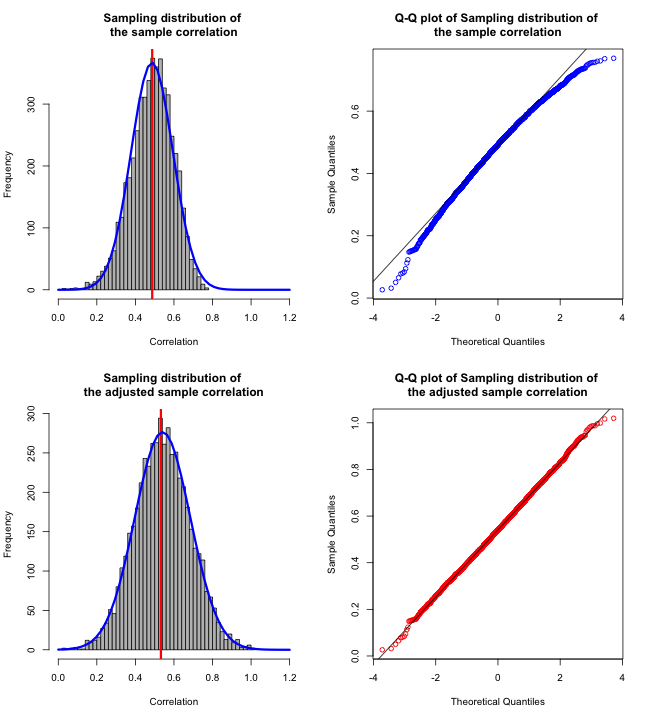Red vertical lines represent regular and adjuster correlation coefficients in the population. Using Q-Q plot we can visually inspect deviations from the normal curve (adjusted has very small deviation compared to normal)

With adjusted correlation coefficient we can calculate SE from formula, and because it is normaly distributed we can calculate probabilities from normal distribution using Z-scores.

Formula for standard error (SE) for adjusted correlation coefficient is

SE = 1 / SQRT( N - 3 )

According to this formula, SE for our population is 0.15 when N=50. The standard deviation of simulated distribution of adjusted sample correlation is 0.14, which is very close. So the formula is correct.

Once we are done with the statistical tests or calculation of confidence intervals (more in a minute) we can re-adjust the coefficient to the normal one. Hence, we are using adjusted correlation coefficient for his SE normal distribution to perform statistical tests. Once we are done with that we convert it to the original. Make sense?

## Statistical significance and Null Hypothesis Testing

Knowing standard error (SE) in the population we can calculate probability of acquiring certain sample result. The only problem is that we don't know the population SE. The only option we can do is to use sample SE.

What we have in real life/study (without knowing the whole population) is

• Adjusted Correlation in the sample
• Standard error of the sample

We know that in the population, adjusted sampling correlation is distributed normaly around mean equal to population correlation with standard deviation equal to standard error (SE).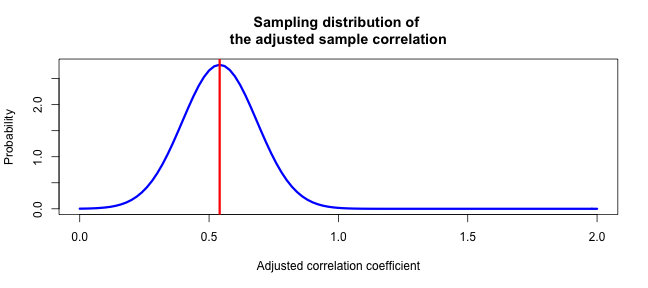We can check if the sample adjusted correlation is statistically significant by utilizing Null Hypothesis Testing (NHT) and calculating p-value. How does this work?

We assume that there is no correlation in the population (r=0). This is called Null Hypothesis. We know that the standard error of null hypothesis (r=0) is equal to standard error of our sample.

What we are interested into is the probability of acquiring score (sample correlation) at least extreme as we did assuming that null hypothesis is true (population r=0).

On the following picture, Null Hypothesis (r=0) is depicted as blue, and the sample correlation we might got is depicted as red line.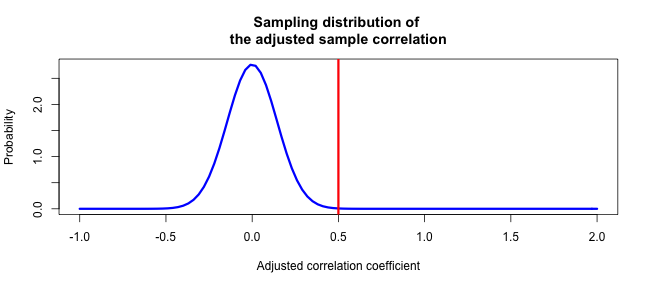Using two-tail test, we can calculate the probability of acquiring score at least as extreme as we did, assuming the Null is true. This called p-value. Since we know the distribution and mean of Null, we can calculate p-value using simple Z-Test (that gives us Z-Statistic or Z-Number). Please note that probability is surface under curve.

Let's take one sample and calculate p-value

``````sampleSize <- 10

sample <- population[sample(nrow(population), sampleSize), ]

sampleCorrelation <- cor(sample\$squat, sample\$verticalJump)

sampleAdjustedCorrelation <- 0.5 * log((1 + sampleCorrelation)/(1 - sampleCorrelation))

standardError <- 1/sqrt(sampleSize - 3)

pValue <- (1 - pnorm(Z.Statistic, mean = 0, sd = standardError)) * 2
``````

This is what we got

• r= 0.38
• SE= 0.38
• p-value= 0.0046

If p-value equals to zero, that means it is so low that it is beyond R's calculation power.

Researchers usually take 0.05 as Alpha level to accept statistical significance and reject null. So if p-value < 0.05 then we reject null hypothesis and say that we are >95% certain that there is a effect in the population (in this case correlation in the population)

BTW, in the calculus above I should have used T-Test and T-Statistic instead of Z-test, but for the sake of simplicity I took Z-score. The calculus using T-Test is a bit different. One could use R function cor.test

``````correlationTest <- cor.test(sample\$squat, sample\$verticalJump)

print(correlationTest)
``````
``````##
##  Pearson's product-moment correlation
##
## data:  sample\$squat and sample\$verticalJump
## t = 1.176, df = 8, p-value = 0.2733
## alternative hypothesis: true correlation is not equal to 0
## 95 percent confidence interval:
##  -0.3239  0.8163
## sample estimates:
##   cor
## 0.384
``````

Using cor.test we get p-value of 0.2733.

## Dance of p-values

What happens with p-value if we repeat the sampling numerous times for different sample sizes? Let's find out.

``````samplingNumber <- 5000

samplePValue <- rep(0, samplingNumber)

par(mfrow = c(3, 3))
for (sampleSize in c(3, 5, 10, 15, 20, 25, 30, 35, 40)) {

for (i in seq(from = 1, to = samplingNumber)) {
# take sample from population
sample <- population[sample(nrow(population), sampleSize), ]

# remember the p-value
samplePValue[i] <- cor.test(sample\$squat, sample\$verticalJump)\$p.value
}
# Plot the p-value distribution
hist(samplePValue, 50, col = "blue", main = paste("N=", round(sampleSize,
0)), xlab = "p-value")
abline(v = 0.05, col = "red", lwd = 3)
}
``````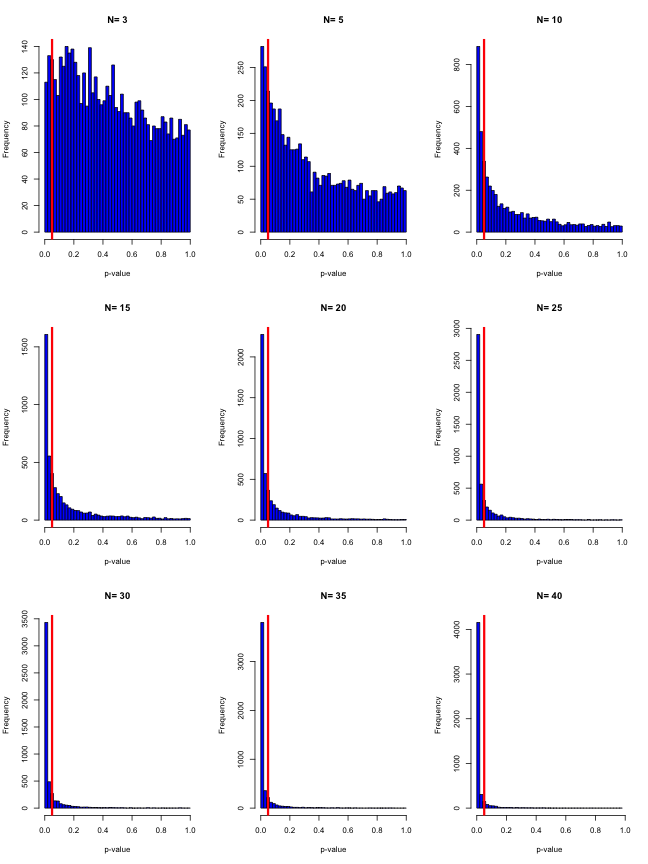The red line represent 0.05 cut-off (alpha) for statistical significance.As you can see the distribution of p=values depends a lot on sample size. Bigger the sample the higher the chance to get statistical significance score. You can find more about it in this video by Geoff Cumming

Another way to represent inferences to a population are confidence intervals, and in my opinion and opinion of many others, are much better way than p-values. I might cover confidence intervals in some future posts.

#### 1 comment:

1.Wow, great stuff, Will is the supervisor for my Master's research project. I am using R as well and looking to get up to speed with it as well. All the best. Hamish